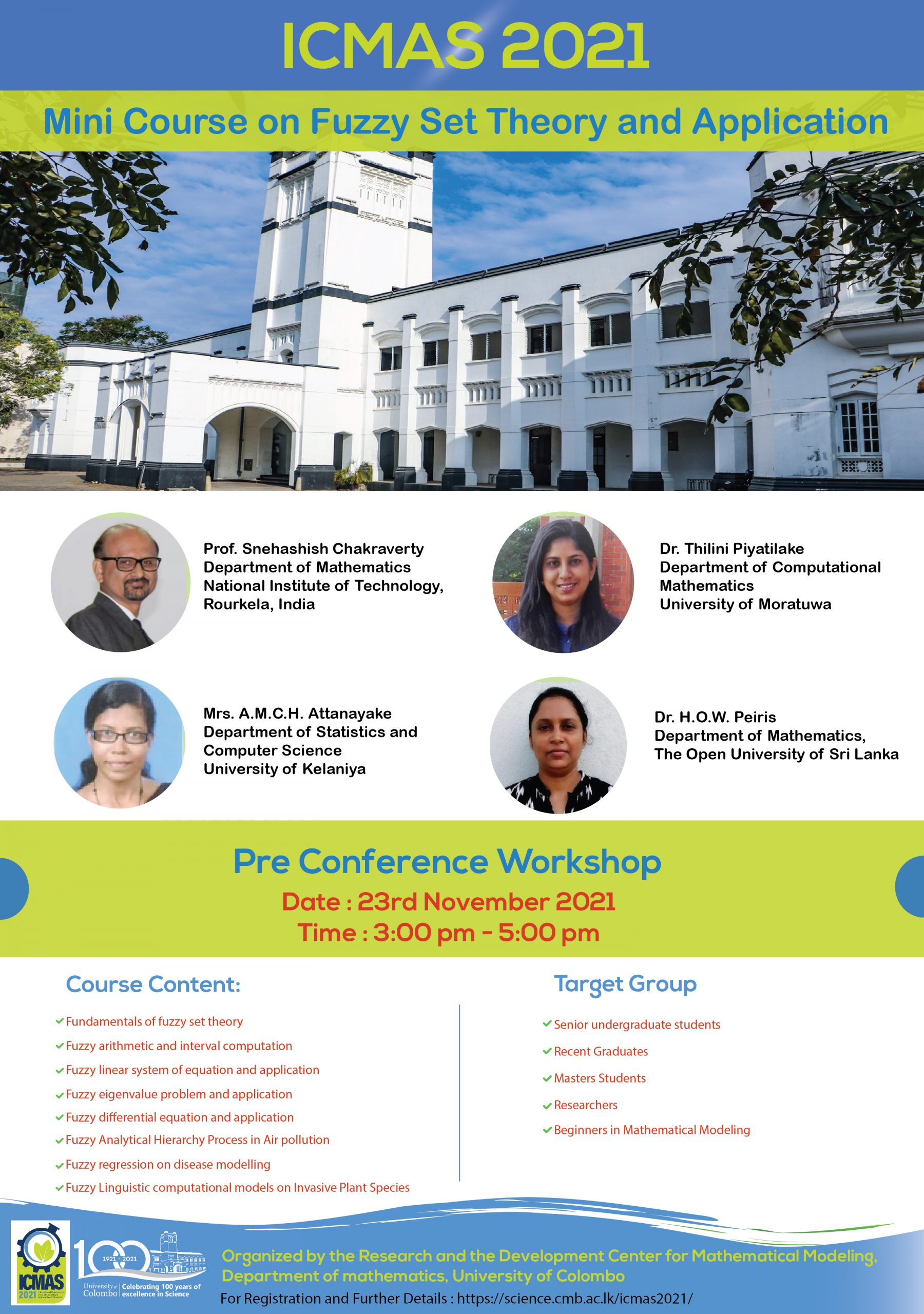## Mini-course on Fuzzy Set Theory and Applications

Organized by the Research and Development Center for Mathematical Modeling, Department of Mathematics, University of Colombo

In this workshop, basics of fuzzy set theory and related arithmetic will be discussed. In this regard, fuzzy system of equations, fuzzy eigenvalue problems and fuzzy differential equations will be introduced in brief. Further, few challenging interdisciplinary problems will be addressed in fuzzy uncertain scenarios. The target of the session is to meet the present and future needs for the interaction between various science and technology/engineering areas on the one hand and different types of fuzzy computing on the other.

Date : 23rd November 2021

Time: 3:00 pm – 5:00 pm (IST)

### Course content

• Fundamentals of fuzzy set theory
• Fuzzy arithmetic and interval computation
• Fuzzy linear system of equation and application
• Fuzzy eigenvalue problem and application
• Fuzzy differential equation and application
• Fuzzy Analytical Hierarchy Process in Air pollution
• Fuzzy regression on Disease modelling
• Fuzzy Linguistic computational models on Invasive Plant Species

### Target Group

• Masters Students
• Researchers
• Beginners in Mathematical Modeling

### Resource Persons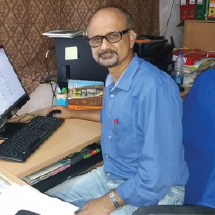##### Prof. Snehashish Chakraverty

Department of Mathematics
National Institute of Technology,
Rourkela, India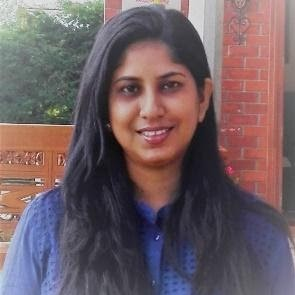##### Dr. Thilini Piyatilake

Department of Computational Mathematics
University of Moratuwa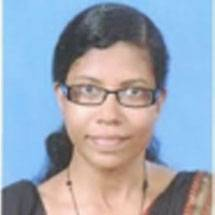##### Mrs. A.M.C.H. Attanayake

Department of Statistics and Computer Science
University of Kelaniya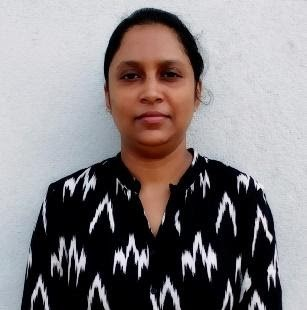##### Dr. H.O.W. Peiris

Department of Mathematics,
The Open University of Sri Lanka